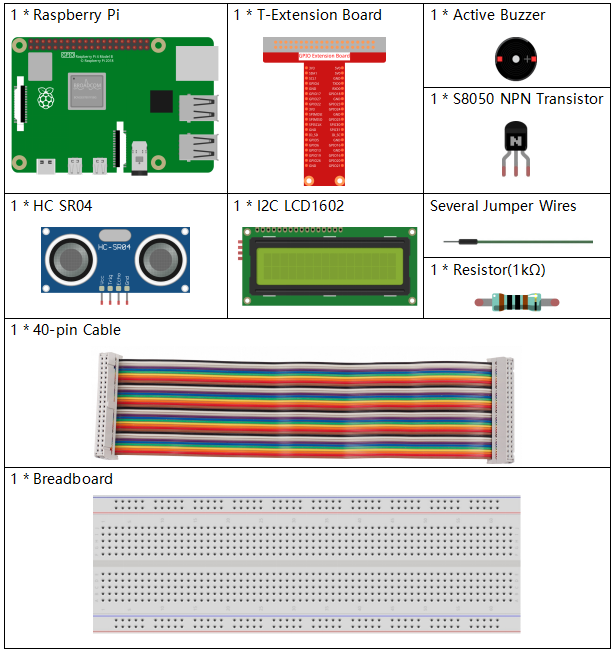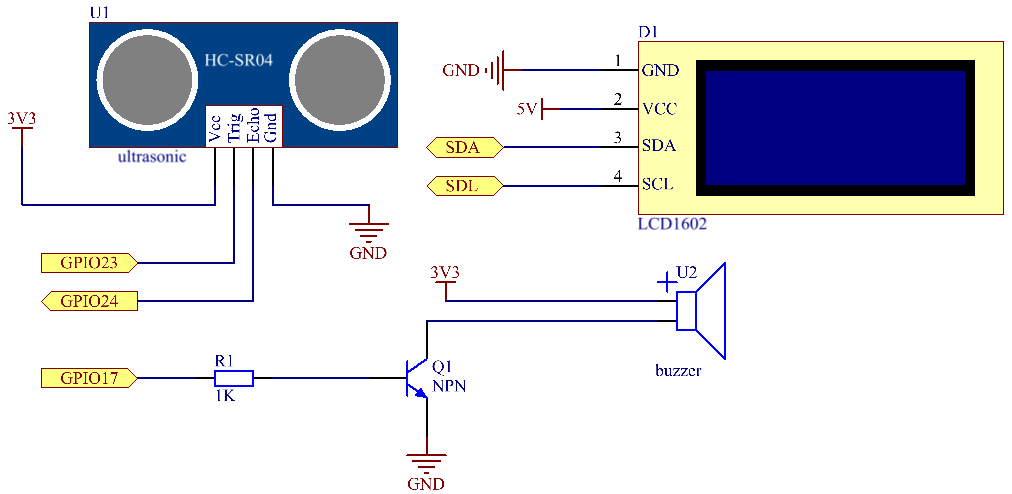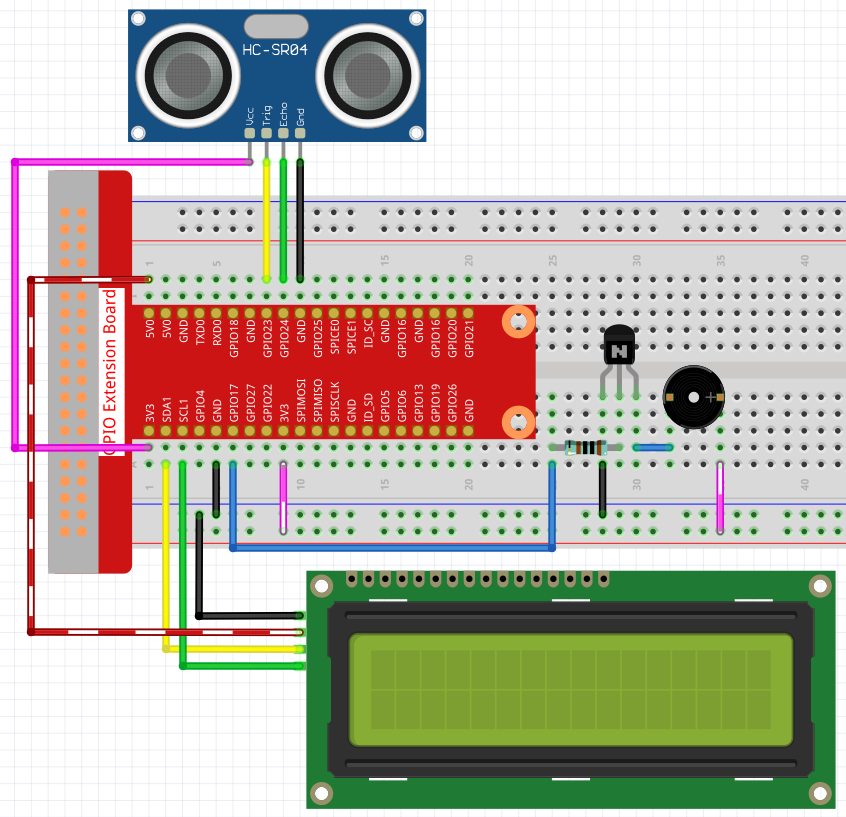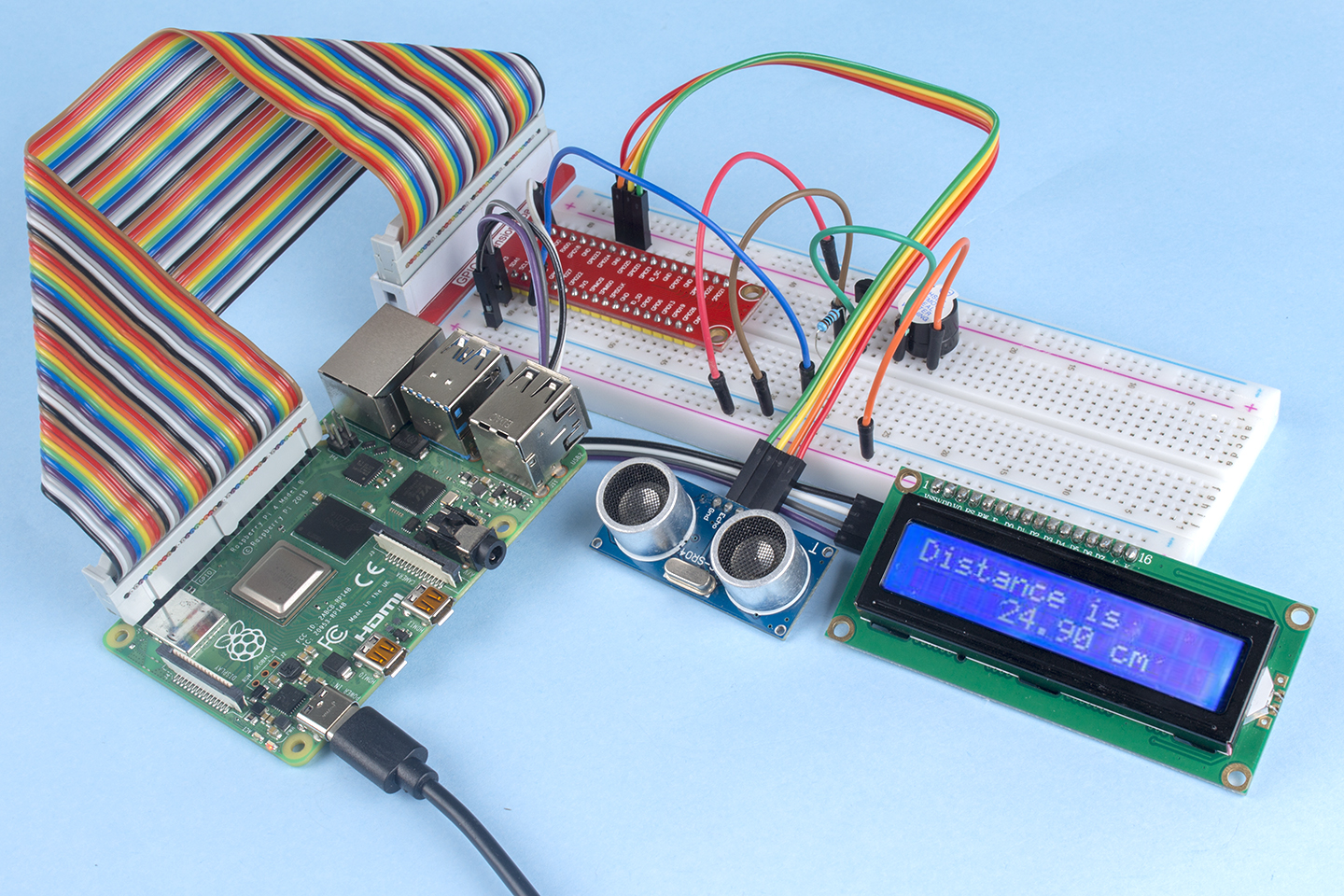# 3.1.3 Reversing Alarm¶

## Introduction¶

In this project, we will use LCD, buzzer and ultrasonic sensors to make a reverse assist system. We can put it on the remote control vehicle to simulate the actual process of reversing the car into the garage.

## Components¶## Schematic Diagram¶

Ultrasonic sensor detects the distance between itself and the obstacle that will be displayed on the LCD in the form of code. At the same time, the ultrasonic sensor let the buzzer issue prompt sound of different frequency according to different distance value.

 T-Board Name physical wiringPi BCM GPIO23 Pin 16 4 23 GPIO24 Pin 18 5 24 GPIO17 Pin 11 0 17 SDA1 Pin 3 SCL1 Pin 5## Experimental Procedures¶

Step 1: Build the circuit.### For C Language Users¶

Step 2: Change directory.

```cd /home/pi/davinci-kit-for-raspberry-pi/c/3.1.3/
```

Step 3: Compile.

```gcc 3.1.3_ReversingAlarm.c -lwiringPi
```

Step 4: Run.

```sudo ./a.out
```

As the code runs, ultrasonic sensor module detects the distance to the obstacle and then displays the information about the distance on LCD1602; besides, buzzer emits warning tone whose frequency changes with the distance.

Note

If it does not work after running, or there is an error prompt: “wiringPi.h: No such file or directory”, please refer to C code is not working?.

Code

Note

The following codes are incomplete. If you want to check the complete codes, you are suggested to use command nano 3.1.1_ReversingAlarm.c.

```#include <wiringPi.h>
#include <stdio.h>
#include <sys/time.h>
#include <wiringPi.h>
#include <wiringPiI2C.h>
#include <string.h>

#define Trig    4
#define Echo    5
#define Buzzer  0

int BLEN = 1;
int fd;

//here is the function of LCD
void write_word(int data){...}

void send_command(int comm){...}

void send_data(int data){...}

void lcdInit(){...}

void clear(){...}

void write(int x, int y, char data[]){...}

//here is the function of Ultrasonic
void ultraInit(void){...}

float disMeasure(void){...}

//here is the main function
int main(void)
{
float dis;
char result;
if(wiringPiSetup() == -1){
printf("setup wiringPi failed !");
return 1;
}

pinMode(Buzzer,OUTPUT);
lcdInit();
ultraInit();

clear();
write(0, 0, "Ultrasonic Starting");
write(1, 1, "By Sunfounder");

while(1){
dis = disMeasure();
printf("%.2f cm \n",dis);
delay(100);
digitalWrite(Buzzer,LOW);
if (dis > 400){
clear();
write(0, 0, "Error");
write(3, 1, "Out of range");
delay(500);
}
else
{
clear();
write(0, 0, "Distance is");
sprintf(result,"%.2f cm",dis);
write(5, 1, result);

if(dis>=50)
{delay(500);}
else if(dis<50 & dis>20) {
for(int i=0;i<2;i++){
digitalWrite(Buzzer,HIGH);
delay(50);
digitalWrite(Buzzer,LOW);
delay(200);
}
}
else if(dis<=20){
for(int i=0;i<5;i++){
digitalWrite(Buzzer,HIGH);
delay(50);
digitalWrite(Buzzer,LOW);
delay(50);
}
}
}
}

return 0;
}
```

Code Explanation

```pinMode(Buzzer,OUTPUT);
lcdInit();
ultraInit();
```

In this program, we apply previous components synthetically. Here we use buzzers, LCD and ultrasonic. We can initialize them the same way as we did before.

```dis = disMeasure();
printf("%.2f cm \n",dis);
digitalWrite(Buzzer,LOW);
if (dis > 400){
write(0, 0, "Error");
write(3, 1, "Out of range");
}
else
{
write(0, 0, "Distance is");
sprintf(result,"%.2f cm",dis);
write(5, 1, result);
}
```

Here we get the value of the ultrasonic sensor and get the distance through calculation.

If the value of distance is greater than the range value to be detected, an error message is printed on the LCD. And if the distance value is within the range, the corresponding results will be output.

```sprintf(result,"%.2f cm",dis);
```

Since the output mode of LCD only supports character type, and the variable dis stores the value of float type, we need to use sprintf(). The function converts the float type value to a character and stores it on the string variable result[]. %.2f means to keep two decimal places.

```if(dis>=50)
{delay(500);}
else if(dis<50 & dis>20) {
for(int i=0;i<2;i++){
digitalWrite(Buzzer,HIGH);
delay(50);
digitalWrite(Buzzer,LOW);
delay(200);
}
}
else if(dis<=20){
for(int i=0;i<5;i++){
digitalWrite(Buzzer,HIGH);
delay(50);
digitalWrite(Buzzer,LOW);
delay(50);
}
}
```

This judgment condition is used to control the sound of the buzzer. According to the difference in distance, it can be divided into three cases, in which there will be different sound frequencies. Since the total value of delay is 500, all of the cases can provide a 500ms interval for the ultrasonic sensor.

### For Python Language Users¶

Step 2: Change directory.

```cd /home/pi/davinci-kit-for-raspberry-pi/python/
```

Step 3: Run.

```sudo python3 3.1.3_ReversingAlarm.py
```

As the code runs, ultrasonic sensor module detects the distance to the obstacle and then displays the information about the distance on LCD1602; besides, buzzer emits warning tone whose frequency changes with the distance.

Code

Note

You can Modify/Reset/Copy/Run/Stop the code below. But before that, you need to go to source code path like `davinci-kit-for-raspberry-pi/python`.

```import LCD1602
import time
import RPi.GPIO as GPIO

TRIG = 16
ECHO = 18
BUZZER = 11

def lcdsetup():
LCD1602.init(0x27, 1)   # init(slave address, background light)
LCD1602.clear()
LCD1602.write(0, 0, 'Ultrasonic Starting')
LCD1602.write(1, 1, 'By SunFounder')
time.sleep(2)

def setup():
GPIO.setmode(GPIO.BOARD)
GPIO.setup(TRIG, GPIO.OUT)
GPIO.setup(ECHO, GPIO.IN)
GPIO.setup(BUZZER, GPIO.OUT, initial=GPIO.LOW)
lcdsetup()

def distance():
GPIO.output(TRIG, 0)
time.sleep(0.000002)

GPIO.output(TRIG, 1)
time.sleep(0.00001)
GPIO.output(TRIG, 0)

while GPIO.input(ECHO) == 0:
a = 0
time1 = time.time()
while GPIO.input(ECHO) == 1:
a = 1
time2 = time.time()

during = time2 - time1
return during * 340 / 2 * 100

def destroy():
GPIO.output(BUZZER, GPIO.LOW)
GPIO.cleanup()
LCD1602.clear()

def loop():
while True:
dis = distance()
print (dis, 'cm')
print ('')
GPIO.output(BUZZER, GPIO.LOW)
if (dis > 400):
LCD1602.clear()
LCD1602.write(0, 0, 'Error')
LCD1602.write(3, 1, 'Out of range')
time.sleep(0.5)
else:
LCD1602.clear()
LCD1602.write(0, 0, 'Distance is')
LCD1602.write(5, 1, str(round(dis,2)) +' cm')
if(dis>=50):
time.sleep(0.5)
elif(dis<50 and dis>20):
for i in range(0,2,1):
GPIO.output(BUZZER, GPIO.HIGH)
time.sleep(0.05)
GPIO.output(BUZZER, GPIO.LOW)
time.sleep(0.2)
elif(dis<=20):
for i in range(0,5,1):
GPIO.output(BUZZER, GPIO.HIGH)
time.sleep(0.05)
GPIO.output(BUZZER, GPIO.LOW)
time.sleep(0.05)

if __name__ == "__main__":
setup()
try:
loop()
except KeyboardInterrupt:
destroy()
```

Code Explanation

```def lcdsetup():
LCD1602.init(0x27, 1)   # init(slave address, background light)

def setup():
GPIO.setmode(GPIO.BOARD)
GPIO.setup(TRIG, GPIO.OUT)
GPIO.setup(ECHO, GPIO.IN)
GPIO.setup(BUZZER, GPIO.OUT, initial=GPIO.LOW)
lcdsetup()
```

In this program, we apply the previously used components synthetically. Here we use buzzers, LCD and ultrasonic. We can initialize them in the same way as we did before.

```dis = distance()
print (dis, 'cm')
print ('')
GPIO.output(BUZZER, GPIO.LOW)
if (dis > 400):
LCD1602.clear()
LCD1602.write(0, 0, 'Error')
LCD1602.write(3, 1, 'Out of range')
time.sleep(0.5)
else:
LCD1602.clear()
LCD1602.write(0, 0, 'Distance is')
LCD1602.write(5, 1, str(round(dis,2)) +' cm')
```

Here we get the values of the ultrasonic sensor and get the distance through calculation. If the value of distance is greater than the range of value to be detected, an error message is printed on the LCD. And if the distance is within the working range, the corresponding results will be output.

LCD1602.write(5, 1, str(round(dis,2)) +’ cm’)

Since the LCD output only supports character types, we need to use str () to convert numeric values to characters. We are going to round it to two decimal places.

```if(dis>=50)
{delay(500);}
else if(dis<50 & dis>20) {
for(int i=0;i<2;i++){
digitalWrite(Buzzer,HIGH);
delay(50);
digitalWrite(Buzzer,LOW);
delay(200);
}
}
else if(dis<=20){
for(int i=0;i<5;i++){
digitalWrite(Buzzer,HIGH);
delay(50);
digitalWrite(Buzzer,LOW);
delay(50);
}
}
```

This judgment condition is used to control the sound of the buzzer. According to the difference in distance, it can be divided into three cases, in which there will be different sound frequencies. Since the total value of delay is 500, all of them can provide a 500ms interval for the ultrasonic sensor to work.

## Phenomenon Picture¶